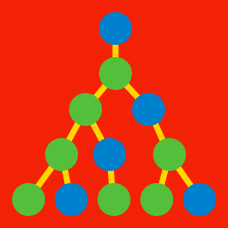Discrete Mathematics

Linear Recurrence Relations - Basic Substitutions

The sequence $\{x_n\}$ satisfies the relation $\begin{array}{c}&x_1 = 5,&x_{n+1} = x_n + 5 &(n = 1, 2, 3, \ldots). \end{array}$ What is the value of $x_{20}?$

The sequence $\{x_n\}$ satisfies the relation $\begin{array}{c}&x_1 = 2, &x_{n+1} = 2 x_n + 1 &(n = 1, 2, 3, \ldots). \end{array}$ What is the value of $x_{5}?$

The sequence $\{x_n\}$ satisfies the relation $\begin{array}{c}&x_1 = 1, &x_2 = 5, &x_{n+2} = 4 x_{n+1} + 5 x_n &(n = 1, 2, 3, \ldots). \end{array}$ What is the value of $\dfrac{x_8}{625}?$

The sequence $\{x_n\}$ satisfies the relation $\begin{array}{c}&x_1 = 1, &x_2 = 0, &6x_{n+2} = 5 x_{n+1} - x_n &(n = 1, 2, 3, \cdots). \end{array}$ What is the value $n$ that satisfies $x_n = \dfrac{1}{3^{10}} - \dfrac{1}{2^{10}}?$

The sequence $\{x_n\}$ satisfies the relation $\begin{array}{c}&x_1 = 4, &x_{n+1} = x_n + 3n - 2 &(n = 1, 2, 3, \ldots). \end{array}$ What is the value of $x_{12}?$

×